#Wolfram Summer School

# Alumni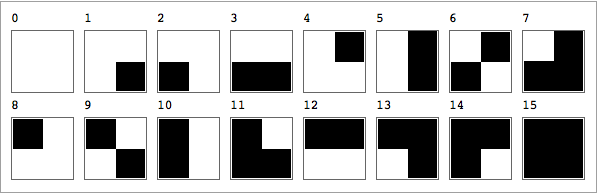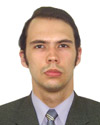We consider 2-dimensional block cellular automata (BCA) with intention to meet simple physical requirements such as reversibility and space isotropy. Along with general study of the whole class of 2×2-neighborhood BCA, several rule subspaces that meet physical criteria are studied. It is also interesting to examine the possibility of the energy conservation expressed in terms of local rules. The energy function is set by the Ising model. Interpretation of domain, oscillating, particle and other patterns in terms of physical phenomena were studied. Some statements about impossibility of energy conservation laws expressed by 2D 2×2 BCA local rules were proved and verified experimentally with filtration methods.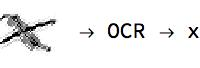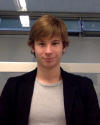This project aims at developing a useful optical character recognition by means of a neural network. It is planned that the network will be able to understand every glyph in the world—that is, when a glyph is given as input, the net should give the correct character as output. For that, a set containing all glyphs should be provided so that we can apply many image transformations, like those in images, to train the net with a very comprehensive training set.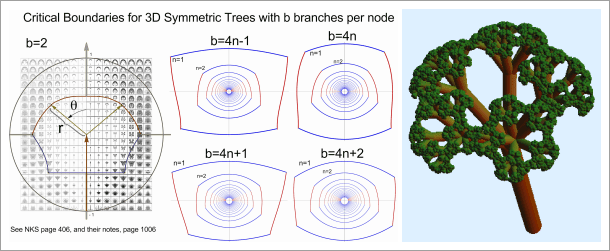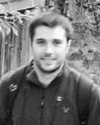I identified four kinds of symmetric fractal trees in 3D that are determined by the type of expressions found in their boundary equations; these are trees with number of branches b=4n-1, b=4n, b=4n+1 and b=4n+2 where n takes the integer values from 1 to ∞. Several animations were produced when one walks around the critical boundaries of the parameter space, showing interesting dynamics and topological critical changes for certain angles.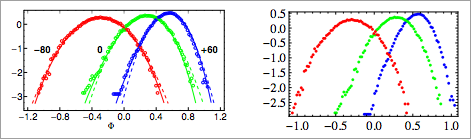My project consisted of a set of functions that can extract a plot dataset when provided the vector representation of the plot. I have been able to successfully identify axes, ticks, labels and data points for my self-generated plots. In this situation the error is negligible and depends mainly on a geometric object with nonzero area used to identify the points in the set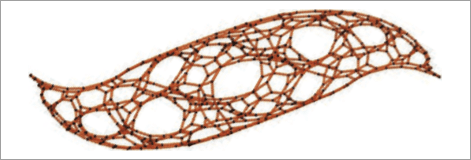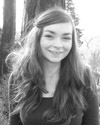The aim of this project is to identify the manifolds corresponding to networks that are generated by simple substitution rules from Stephen Wolfram’s physics project. At first glance, some of the networks resemble a cell complex of a known surface. The idea is to provide techniques to substantiate this impression and figure out the geometric structure that might underlie the purely combinatorially given network.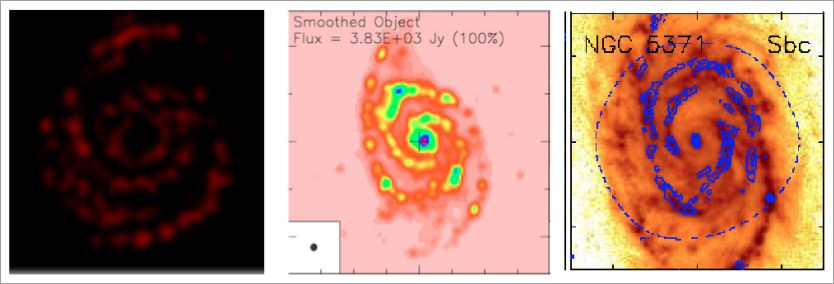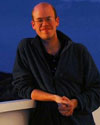We propose to explore a number of analytical galaxy rotation curves to create a template library of cellular automata galaxies. A quantitative measure of galaxy morphology needs to be ascertained to compare observations (perhaps at multiple wavelengths) to the CA galaxies. Polar basis functions consisting of Chebyshev rational functions TLn(r) and Fourier series (Jimenez-Teja & Benitez 2011) can be applied to both the archival survey imaging as well as the simulations. The basis coefficients for observations and models can be used as a figure of merit or metric for comparison, matching CA simulation galaxies to sets of cataloged images via the parameterized effective radius.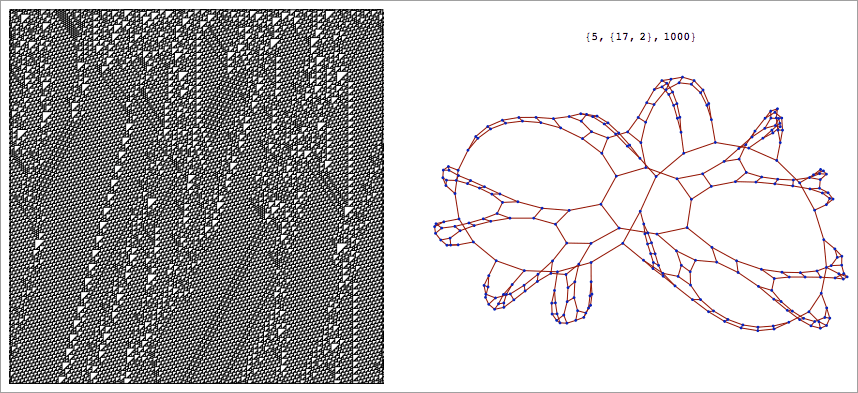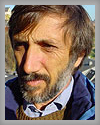We have devised a simple way to introduce temporary perturbations in trinet computations and have visualized their effects by the revisit indicator, which turns out to be especially useful for this purpose. This technique can indeed be used also for exploring behaviors starting from random initial conditions.